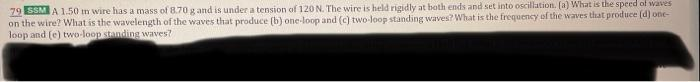Home / Expert Answers / Physics / 79-ssm-a-1-50-mathrm-tr-wire-has-a-mass-of-8-70-mathrm-g-and-is-under-a-tension-of-pa978

# (Solved): 79 SSM A $$1.50 \mathrm{tr}$$ wire has a mass of $$8.70 \mathrm{~g}$$ and is under a tension of ...79 SSM A $$1.50 \mathrm{tr}$$ wire has a mass of $$8.70 \mathrm{~g}$$ and is under a tension of $$120 \mathrm{~N}$$. The wire is held rigidly at beth ends and set into oscillation. (a) What is the speed of waves on the wire? What is the wavelength of the waves that produce (b) one-loop and (c) two-loop standing waves? What is the freguency of the werves that produce (d) obeloop and $$(e)$$ two-loop standing waves?

We have an Answer from Expert